# Elm – fun with L-System (Part 5)

Series:

1. Algae
2. Pythago­ras Tree
3. Can­tor Dust
4. Koch Curve
5. Sier­pin­ski Triangle (this)
6. Dragon Curve
7. Frac­tal Plant

Last time out we imple­mented the Koch Curve exam­ple from the L-System wikipedia page. Now, let’s con­tinue our jour­ney and see how we can imple­ment the Sierpinski Triangle exam­ple in Elm.

## Example 5 : Sierpinski Triangle

First, let’s define our L-System: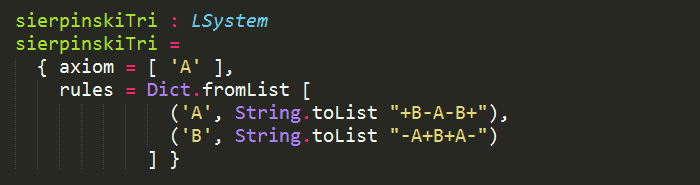Since we have done a lot of the heavy lifting in the last post (recall the Path module we created), we actually don’t have much to do here.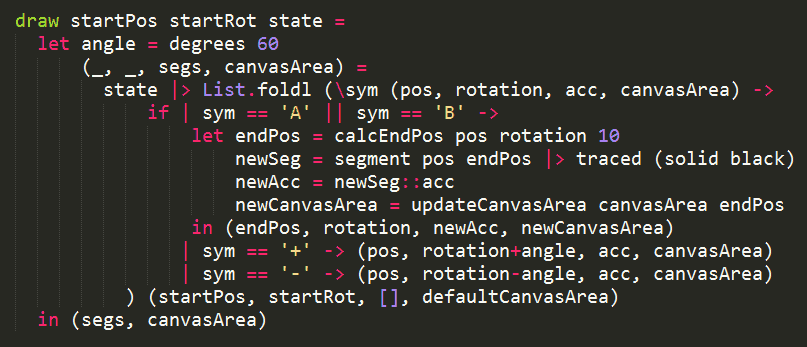The only thing of interest here is that both ‘A’ and ‘B’ mean “draw forward”, but need to be represented different for the growth rules.

As far as I know, (please correct me in the comments if I’m wrong about this) there is no way to handle both symbols using case..of here, e.g.

case sym of

‘A’ | ‘B’ –> …

similar to how one would do in F#:

match sym of

| ‘A’ | ‘B’ –> …

| ‘+’ –> …

| ‘–‘ –> …

Hence why I opted for a multi-way if statement here instead.

Here’s how it looks, feel free to try it out yourself via the live demo links below.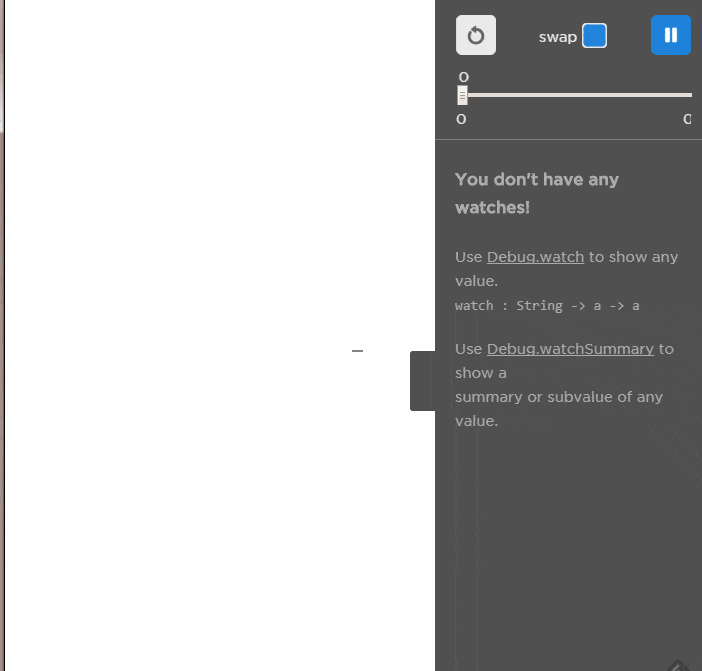But wait, the L-System wikipedia page also mentions a second way to draw the Sierpinski Triangle: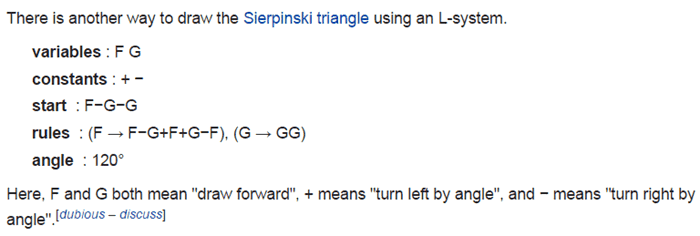So, let’s implemented this as well, as v2.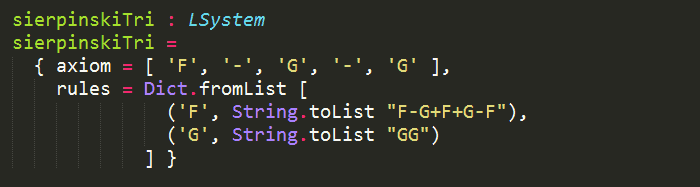When I used the suggested 120 degrees, I got an upside-down triangle. So instead, I used –120 degrees here: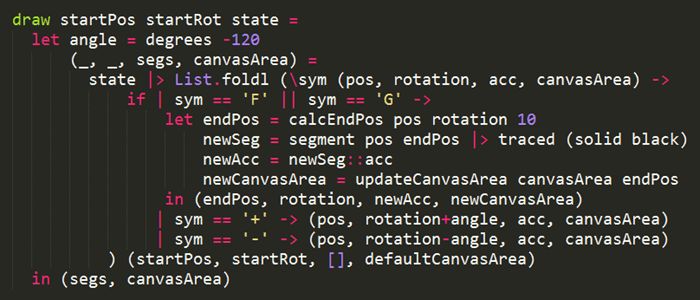Yup, it’s basically a carbon copy of the first version, except for a different value for angle  plus different symbols.

Why are the examples not consistent with the choice of symbols for “draw forward”? One wonders…

Anyhow, here’s how it looks, which you can also try out via the live demo links below.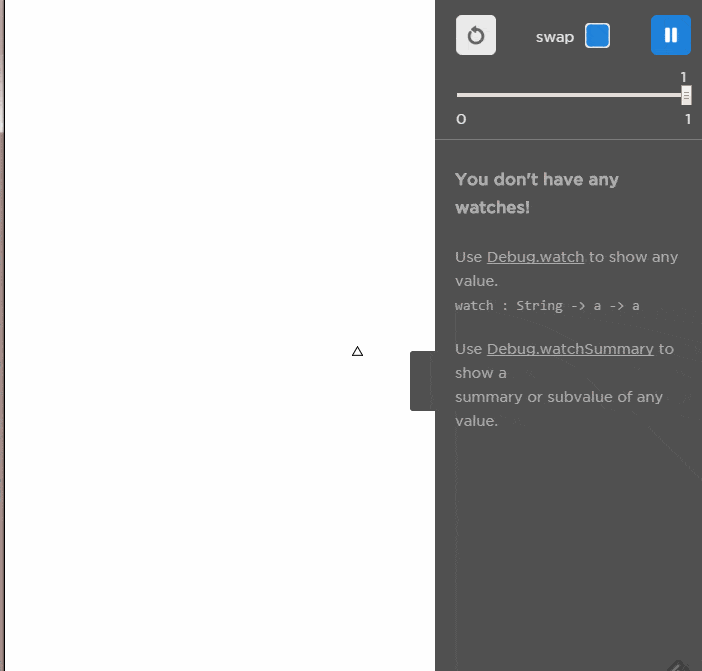## Live Demo (here, here)

Use LEFT and RIGHT arrow keys to evolve/devolve the L-System.

## Source Code (here, here)

Next : Dragon Curve